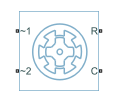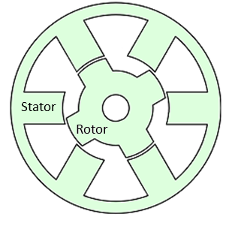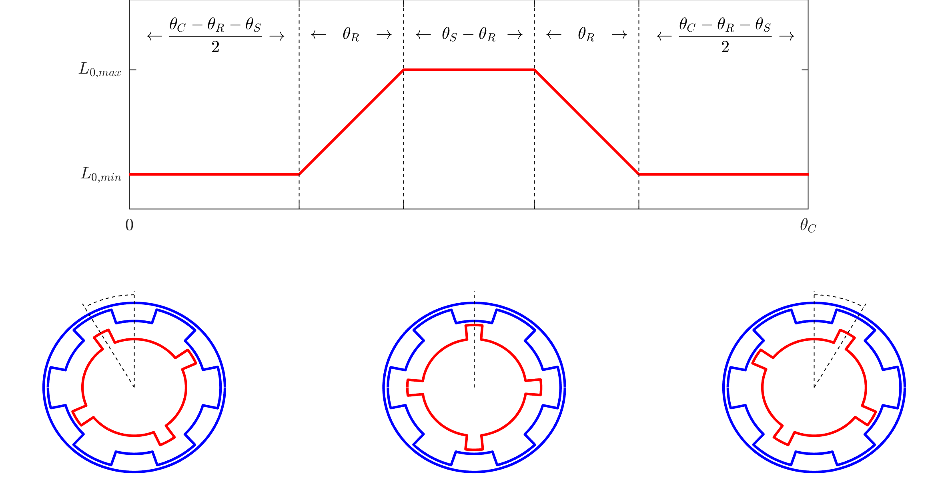# Switched Reluctance Machine

Three-phase switched reluctance machine

•Libraries:
Simscape / Electrical / Electromechanical / Reluctance & Stepper

## Description

The Switched Reluctance Machine block represents a three-phase switched reluctance machine (SRM). The stator has three pole pairs, carrying the three motor windings, and the rotor has several nonmagnetic poles. The motor produces torque by energizing a stator pole pair, inducing a force on the closest rotor poles and pulling them toward alignment. The diagram shows the motor construction.Choose this machine in your application to take advantage of these properties:

• Low cost

• Relatively safe failing currents

• Robustness to high temperature operation

• High torque-to-inertia ratio

Use this block to model an SRM using easily measurable or estimable parameters. To model an SRM using FEM data, see Switched Reluctance Motor Parameterized with FEM Data.

### Equations

Switched Reluctance Machine Block

The rotor stroke angle for a three-phase machine is

`${\theta }_{st}=\frac{2\pi }{3{N}_{r}},$`

where:

• θst is the stoke angle.

• Nr is the number of rotor poles.

The torque production capability, β, of one rotor pole is

`$\beta =\frac{2\pi }{{N}_{r}}.$`

The mathematical model for a switched reluctance machine (SRM) is highly nonlinear due to influence of the magnetic saturation on the flux linkage-to-angle, λ(θph) curve. The phase voltage equation for an SRM is

`${v}_{ph}={R}_{s}{i}_{ph}+\frac{d{\lambda }_{ph}\left({i}_{ph},{\theta }_{ph}\right)}{dt}$`

where:

• vph is the voltage per phase.

• Rs is the stator resistance per phase.

• iph is the current per phase.

• λph is the flux linkage per phase.

• θph is the angle per phase.

Rewriting the phase voltage equation in terms of partial derivatives yields this equation:

`${v}_{ph}={R}_{s}{i}_{ph}+\frac{\partial {\lambda }_{ph}}{\partial {i}_{ph}}\frac{d{i}_{ph}}{dt}+\frac{\partial {\lambda }_{ph}}{\partial {\theta }_{ph}}\frac{d{\theta }_{ph}}{dt}.$`

Transient inductance is defined as

`${L}_{t}\left({i}_{ph},{\theta }_{ph}\right)=\frac{\partial {\lambda }_{ph}\left({i}_{ph},{\theta }_{ph}\right)}{\partial {i}_{ph}},$`

or more simply as

`$\frac{\partial {\lambda }_{ph}}{\partial {i}_{ph}}.$`

Back electromotive force is defined as

`${E}_{ph}=\frac{\partial {\lambda }_{ph}}{\partial {\theta }_{ph}}{\omega }_{r}.$`

Substituting these terms into the rewritten voltage equation yields this voltage equation:

`${v}_{ph}={R}_{s}{i}_{ph}+{L}_{t}\left({i}_{ph},{\theta }_{ph}\right)\frac{d{i}_{ph}}{dt}+{E}_{ph}.$`

Applying the co-energy formula to equations for torque,

`${T}_{ph}=\frac{\partial W\left({\theta }_{ph}\right)}{\partial {\theta }_{r}},$`

and energy,

`$W\left({i}_{ph},{\theta }_{ph}\right)=\underset{0}{\overset{{i}_{ph}}{\int }}{\lambda }_{ph}\left({i}_{ph},{\theta }_{ph}\right)d{i}_{ph}$`

yields an integral equation that defines the instantaneous torque per phase, that is,

Integrating over the phases give this equation, which defines the total instantaneous torque for a three-phase SRM:

`$T=\sum _{j=1}^{3}{T}_{ph}\left(j\right).$`

The equation for motion is

`$J\frac{d\omega }{dt}=T-{T}_{L}-{B}_{m}\omega$`

where:

• J is the rotor inertia.

• ω is the mechanical rotational speed.

• T is the rotor torque. For the Switched Reluctance Machine block, torque flows from the machine case (block conserving port C) to the machine rotor (block conserving port R).

• TL is the load torque.

• J is the rotor inertia.

• Bm is the rotor damping.

For high-fidelity modeling and control development, use empirical data and finite element calculation to determine the flux linkage curve in terms of current and angle, that is,

`${\lambda }_{ph}\left({i}_{ph},{\theta }_{ph}\right).$`

For low-fidelity modeling, you can also approximate the curve using analytical techniques. One such technique  uses this exponential function:

`${\lambda }_{ph}\left({i}_{ph},{\theta }_{ph}\right)={\lambda }_{sat}\left(1-{e}^{-{i}_{ph}f\left({\theta }_{ph}\right)}\right),$`

where:

• λsat is the saturated flux linkage.

• f(θr) is obtained by Fourier expansion.

For the Fourier expansion, use the first two even terms of this equation:

`$f\left({\theta }_{ph}\right)=a+b\mathrm{cos}\left({N}_{r}{\theta }_{ph}\right)$`

where a > b,

and

Switched Reluctance Motor Block

The flux linkage curve is approximated based on parametric and geometric data:

`${\lambda }_{ph}\left({i}_{ph},{\theta }_{ph}\right)={\lambda }_{sat}\left(1-{e}^{-{L}_{0}\left(\theta \right){i}_{ph}/{\lambda }_{sat}}\right),$`

where L0 is the unsaturated inductance.

The effects of saturation are more prominent as the product of current and unsaturated inductance approach the saturated flux linkage value. Specify this value using the Saturated flux linkage parameter.

Differentiating the flux equation then gives the winding inductance:

`$L\left({\theta }_{ph}\right)={L}_{0}\left({\theta }_{ph}\right){e}^{\left(-{L}_{0}\left({\theta }_{ph}\right){i}_{ph}/{\lambda }_{sat}\right)}$`

The unsaturated inductance varies between a minimum and maximum value. The minimum value occurs when a rotor pole is directly between two stator poles. The maximum occurs when the rotor pole is aligned with a stator pole. In between these two points, the block approximates the unsaturated inductance linearly as a function of rotor angle. This figure shows the unsaturated inductance as a rotor pole passes over a stator pole.In the figure:

• θR corresponds to the angle subtended by the rotor pole. Set it using the Angle subtended by each rotor pole parameter.

• θS corresponds to the angle subtended by the stator pole. Set it using the Angle subtended by each stator pole parameter.

• θC corresponds to the angle subtended by this full cycle, determined by 2π/2n where n is the number of stator pole pairs.

### Calculating Iron Losses

The Switched Reluctance Machine (Multi-Phase) block implements the iron losses as a reduction in the electrical torque.

If you set the Stator parameterization parameter to either `Specify parametric data` or ```Specify parametric and geometric data```, specify the Magnetizing resistance parameter to model iron losses.

If you set the Stator parameterization parameter to `Specify tabulated flux data`, the block tabulates the losses as the power lost in each stator phase. The losses for each stator only depends on the stator current in that phase, the rotor angle (geometrically shifted to that phase), and the rotor speed. The iron loss power of the rotor is computed as a percentage of the total iron loss power, as specified in the Percentage of total iron losses associated with the rotor parameter.

If you tabulate the losses with current, speed, and angle, the iron loss torque reduces the electrical torque of a generic "A" phase:

`${\tau }_{elecA}=\tau \left({i}_{A},{\theta }_{A}\right)-{\tau }_{loss}\left({i}_{A},w,{\theta }_{A}\right)$`

where ${\tau }_{loss}\left({i}_{A},w,{\theta }_{A}\right)=sign\left(w\right)\frac{{P}_{loss}\left(abs\left({i}_{A}\right),abs\left(w\right),{\theta }_{A}\right)}{abs\left(w\right)+{w}_{min}}$ is the torque loss computed as the mechanical power loss divided by the rotor speed. wmin is the minimum rotor speed and it is equal to `1` `rad/s`. This value prevents a division by zero when the rotor speed is equal to `0`.

If you tabulate the losses in function of only current and speed, the iron loss torque reduces the electrical torque of a generic "A" phase:

`${\tau }_{loss}\left({i}_{A},w\right)=sign\left(w\right)\frac{{P}_{loss}\left(abs\left({i}_{A}\right),abs\left(w\right)\right)}{abs\left(w\right)+{w}_{min}}.$`

The iron losses are applied to the mechanical side as a reduction in torque. If you create the iron loss table, Ploss, from FEM results, the FEM tool might express the power lost as electrical power instead of mechanical power.

The total electrical torque is equal to the sum of the electrical torque of each phase:

`${\tau }_{elec}={\tau }_{elecA}+{\tau }_{elecB}+{\tau }_{elecC}.$`

The total power dissipated is equal to the sum of the iron losses and the copper losses:

`${P}_{dissipated}=\left({\tau }_{lossA}+{\tau }_{lossB}+{\tau }_{lossC}\right)w+{R}_{a}{i}_{a}^{2}+{R}_{b}{i}_{b}^{2}+{R}_{c}{i}_{c}^{2}.$`

Iron losses with thermal ports

If you expose the thermal ports of the block, the power dissipated in each phase act as heat sources in the motor and in the rotor:

`$\begin{array}{l}{P}_{dissA}={R}_{a}{i}_{a}^{2}+{P}_{ironA}\\ {P}_{dissB}={R}_{b}{i}_{b}^{2}+{P}_{ironB}\\ {P}_{dissC}={R}_{c}{i}_{c}^{2}+{P}_{ironC}\\ {P}_{dissR}={P}_{ironR}\end{array}$`

The iron loss in the rotor is equal to a percentage of the total iron losses. This loss remains constant and does not depend on other states:

`${P}_{ironR}=\frac{percen{t}_{rotor}}{100}\left({\tau }_{lossA}+{\tau }_{lossB}+{\tau }_{lossC}\right)w.$`

Then the remaining iron loss is distributed among the different stator phases:

`$\begin{array}{l}{P}_{ironA}=\left(1-\frac{percen{t}_{rotor}}{100}\right){\tau }_{lossA}\text{\hspace{0.17em}}w\\ {P}_{ironB}=\left(1-\frac{percen{t}_{rotor}}{100}\right){\tau }_{lossB}\text{\hspace{0.17em}}w\\ {P}_{ironC}=\left(1-\frac{percen{t}_{rotor}}{100}\right){\tau }_{lossC}\text{\hspace{0.17em}}w\end{array}$`

### Model Thermal Effects

You can expose thermal ports to model the effects of losses that convert power to heat. To expose the thermal ports, set the Modeling option parameter to either:

• `No thermal port` — The block does not contain thermal ports.

• `Show thermal port` — The block contains multiple thermal conserving ports.

For more information about using thermal ports in actuator blocks, see Simulating Thermal Effects in Rotational and Translational Actuators.

### Numerical Smoothing

In practice, magnetic edge effects prevent the inductance from taking a trapezoidal shape as a rotor pole passes over a stator pole. To model these effects, and to avoid gradient discontinuities that hinder solver convergence, the block smooths the gradient ∂L0/∂θ at inflection points. To change the angle over which this smoothing is applied, use the Angle over which flux gradient changes are smoothed parameter.

### Assumptions

The block assumes that a zero rotor angle corresponds to a rotor pole that is aligned perfectly with the a-phase.

### Variables

To set the priority and initial target values for the block variables before simulation, use the Initial Targets section in the block dialog box or Property Inspector. For more information, see Set Priority and Initial Target for Block Variables.

Nominal values provide a way to specify the expected magnitude of a variable in a model. Using system scaling based on nominal values increases the simulation robustness. You can specify nominal values using different sources, including the Nominal Values section in the block dialog box or Property Inspector. For more information, see System Scaling by Nominal Values.

## Ports

### Conserving

expand all

Electrical conserving three-phase port associated with the positive terminals of the stator windings.

#### Dependencies

To enable this port, set Electrical connection to ```Composite three-phase ports```.

Electrical conserving three-phase port associated with the negative terminals of the stator windings.

#### Dependencies

To enable this port, set Electrical connection to ```Composite three-phase ports```.

Electrical conserving port associated with the positive terminal of stator winding a.

#### Dependencies

To enable this port, set Electrical connection to ```Expanded three-phase ports```.

Electrical conserving port associated with the negative terminal of stator winding a.

#### Dependencies

To enable this port, set Electrical connection to ```Expanded three-phase ports```.

Electrical conserving port associated with the positive terminal of stator winding b.

#### Dependencies

To enable this port, set Electrical connection to ```Expanded three-phase ports```.

Electrical conserving port associated with the negative terminal of stator winding b.

#### Dependencies

To enable this port, set Electrical connection to ```Expanded three-phase ports```.

Electrical conserving port associated with the positive terminal of stator winding c.

#### Dependencies

To enable this port, set Electrical connection to ```Expanded three-phase ports```.

Electrical conserving port associated with the negative terminal of stator winding c.

#### Dependencies

To enable this port, set Electrical connection to ```Expanded three-phase ports```.

Mechanical rotational conserving port associated with the rotor.

Mechanical rotational conserving port associated with the stator or casing.

Thermal conserving port associated with stator winding a.

#### Dependencies

To enable this port, set Modeling option to `Show thermal port`.

Thermal conserving port associated with stator winding b.

#### Dependencies

To enable this port, set Modeling option to `Show thermal port`.

Thermal conserving port associated with stator winding c.

#### Dependencies

To enable this port, set Modeling option to `Show thermal port`.

Thermal conserving port associated with the rotor.

#### Dependencies

To enable this port, set Modeling option to `Show thermal port`.

## Parameters

expand all

Whether to enable the thermal ports of the block and model the effects of losses that convert power to heat.

### Main

Whether to have composite or expanded three-phase ports.

Number of rotor poles.

Per-phase resistance of each of the stator windings.

Method for parameterizing the stator.

#### Dependencies

Selecting `Specify parametric data` enables these parameters:

• Magnetizing resistance

• Aligned inductance

• Unaligned inductance

Selecting ```Specify parametric and geometric data``` enables these parameters:

• Magnetizing resistance

• Aligned inductance

• Unaligned inductance

• Angle subtended by each stator pole

• Angle subtended by each rotor pole

• Angle over which flux gradient changes are smoothed

Selecting `Specify tabulated flux data` enables these parameters:

• Current vector, i

• Angle vector, theta

The total magnetizing resistance for each of the phase windings. The default value `inf` indicates that there are no iron losses.

#### Dependencies

This parameter is exposed when you set Stator parameterization to ```Specify parametric data``` or ```Specify parametric and geometric data```.

#### Dependencies

This parameter is exposed when you set Stator parameterization to ```Specify parametric data``` or ```Specify parametric and geometric data```.

The value of this parameter must be greater than the value of the Unaligned inductance parameter.

#### Dependencies

This parameter is exposed when you set Stator parameterization to ```Specify parametric data``` or ```Specify parametric and geometric data```.

The value of this parameter must be less than the value of the Aligned inductance parameter.

#### Dependencies

This parameter is exposed when you set Stator parameterization to ```Specify parametric data``` or ```Specify parametric and geometric data```.

Angle spanned by each stator tooth. This value must be greater than or equal to the value of Angle subtended by each rotor pole.

#### Dependencies

This parameter is exposed when you set Stator parameterization to ```Specify parametric and geometric data```.

Angle spanned by each rotor tooth.

#### Dependencies

This parameter is exposed when you set Stator parameterization to ```Specify parametric and geometric data```.

Angle over which sharp edges in trapezoidal inductance curve are smoothed. This value must be smaller than the value of Angle subtended by each rotor pole.

#### Dependencies

This parameter is exposed when you set Stator parameterization to ```Specify parametric and geometric data```.

Current vector used to identify the flux linkage curve family.

#### Dependencies

This parameter is exposed when you set Stator parameterization to ```Specify tabulated flux data```.

Angle vector used to identify the flux linkage curve family.

#### Dependencies

This parameter is exposed when you set Stator parameterization to ```Specify tabulated flux data```.

#### Dependencies

This parameter is exposed when you set Stator parameterization to ```Specify tabulated flux data```.

### Iron Losses

Whether to enable iron losses modeling.

#### Dependencies

To enable this parameter, set Stator parameterization to ```Specify tabulated flux data```.

Magnetizing resistance. The value must be greater than zero. The default value is `inf`, which implies that there are no iron losses.

#### Dependencies

To enable this parameter, set Stator parameterization to ```Specify parametric data``` or ```Specify parametric and geometric data```.

Vector of rotor speed at which the iron loss is tabulated.

#### Dependencies

To enable this parameter, set Model to either ```Tabulate losses from current and speed``` or ```Tabulate losses from current, speed, and angle```.

Mechanical power lost in the shaft due to iron losses in a particular phase, tabulated as a function of the instantaneous phase current and the rotor speed.

#### Dependencies

To enable this parameter, set Model to ```Tabulate losses from current and speed```.

Mechanical power lost in the shaft due to iron losses in a particular phase, tabulated as a function of the instantaneous phase current, the rotor speed, and the rotor angle.

#### Dependencies

To enable this parameter, set Model to ```Tabulate losses from current, speed, and angle```.

### Mechanical

Inertia of the rotor attached to mechanical translational port R.

Rotary damping.

### Thermal

To enable these parameters, set Modeling option to `Show thermal port`.

Coefficient α in the equation relating resistance to temperature for all three windings, as described in Thermal Model for Actuator Blocks. The default value, `3.93e-3` 1/K, is for copper.

The temperature for which motor parameters are quoted.

The thermal mass value for the a-, b-, and c-windings. The thermal mass is the energy required to raise the temperature by one degree.

The thermal mass of the rotor. The thermal mass is the energy required to raise the temperature of the rotor by one degree.

Percentage of total iron losses associated with the rotor.

 Boldea, I. and S. A. Nasar. Electric Drives, Second Edition. New York: CRC, 2005.

 Ilic'-Spong, M., R. Marino, S. Peresada, and D. Taylor. “Feedback linearizing control of switched reluctance motors.” IEEE Transactions on Automatic Control. Vol. 32, No. 5, 1987, pp. 371–379.